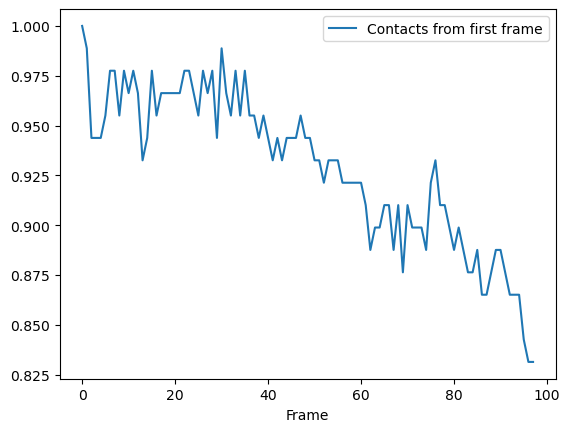# Write your own native contacts analysis method

The contacts.Contacts class has been designed to be extensible for your own analysis. Here we demonstrate how to define a new method to use to determine the fraction of native contacts.

Last updated: December 2022 with MDAnalysis 2.4.0-dev0

Minimum version of MDAnalysis: 1.0.0

Packages required:

:

import MDAnalysis as mda
from MDAnalysis.tests.datafiles import PSF, DCD
from MDAnalysis.analysis import contacts

import numpy as np
import pandas as pd
import matplotlib.pyplot as plt
%matplotlib inline


The test files we will be working with here feature adenylate kinase (AdK), a phosophotransferase enzyme. ([BDPW09]) The trajectory DCD samples a transition from a closed to an open conformation.

:

u = mda.Universe(PSF, DCD)

/home/pbarletta/mambaforge/envs/guide/lib/python3.9/site-packages/MDAnalysis/coordinates/DCD.py:165: DeprecationWarning: DCDReader currently makes independent timesteps by copying self.ts while other readers update self.ts inplace. This behavior will be changed in 3.0 to be the same as other readers. Read more at https://github.com/MDAnalysis/mdanalysis/issues/3889 to learn if this change in behavior might affect you.


## Background

Please see the Fraction of native contacts for an introduction to general native contacts analysis.

## Defining salt bridges

We define salt bridges as contacts between NH/NZ in ARG/LYS and OE*/OD* in ASP/GLU. You may not want to use this definition for real work.

:

sel_basic = "(resname ARG LYS) and (name NH* NZ)"
sel_acidic = "(resname ASP GLU) and (name OE* OD*)"
acidic = u.select_atoms(sel_acidic)
basic = u.select_atoms(sel_basic)


Any function you define must have r and r0 as its first and second arguments respectively, even if you don’t necessarily use them:

• r: an array of distances between atoms at the current time

• r0: an array of distances between atoms in the reference

You can then define following arguments as keyword arguments.

In the function below, we calculate the fraction of native contacts that are less than radius, but greater than min_radius.

:

def fraction_contacts_between(r, r0, radius=3.4, min_radius=2.5):
is_in_contact = (r < radius) & (r > min_radius)  # array of bools
fraction = is_in_contact.sum()/r.size
return fraction


Then we pass fraction_contacts_between to the contacts.Contacts class. Keyword arguments for our custom function must be in the kwargs dictionary. Even though we define a radius keyword in my custom analysis function, it is not automatically passed from contacts.Contacts. We have to make sure that it is in kwargs.

:

ca = contacts.Contacts(u,
select=(sel_acidic, sel_basic),
refgroup=(acidic, basic),
method=fraction_contacts_between,


One easy way to post-process results is to turn them into a dataframe.

:

ca_df = pd.DataFrame(ca.results.timeseries,
columns=['Frame',
'Contacts from first frame'])

:

Frame Contacts from first frame
0 0.0 1.000000
1 1.0 0.988764
2 2.0 0.943820
3 3.0 0.943820
4 4.0 0.943820

### Plotting

We can plot directly from a dataframe (below), or you could use it with other plotting packags such as seaborn.

:

ca_df.plot(x='Frame')

:

<AxesSubplot: xlabel='Frame'>